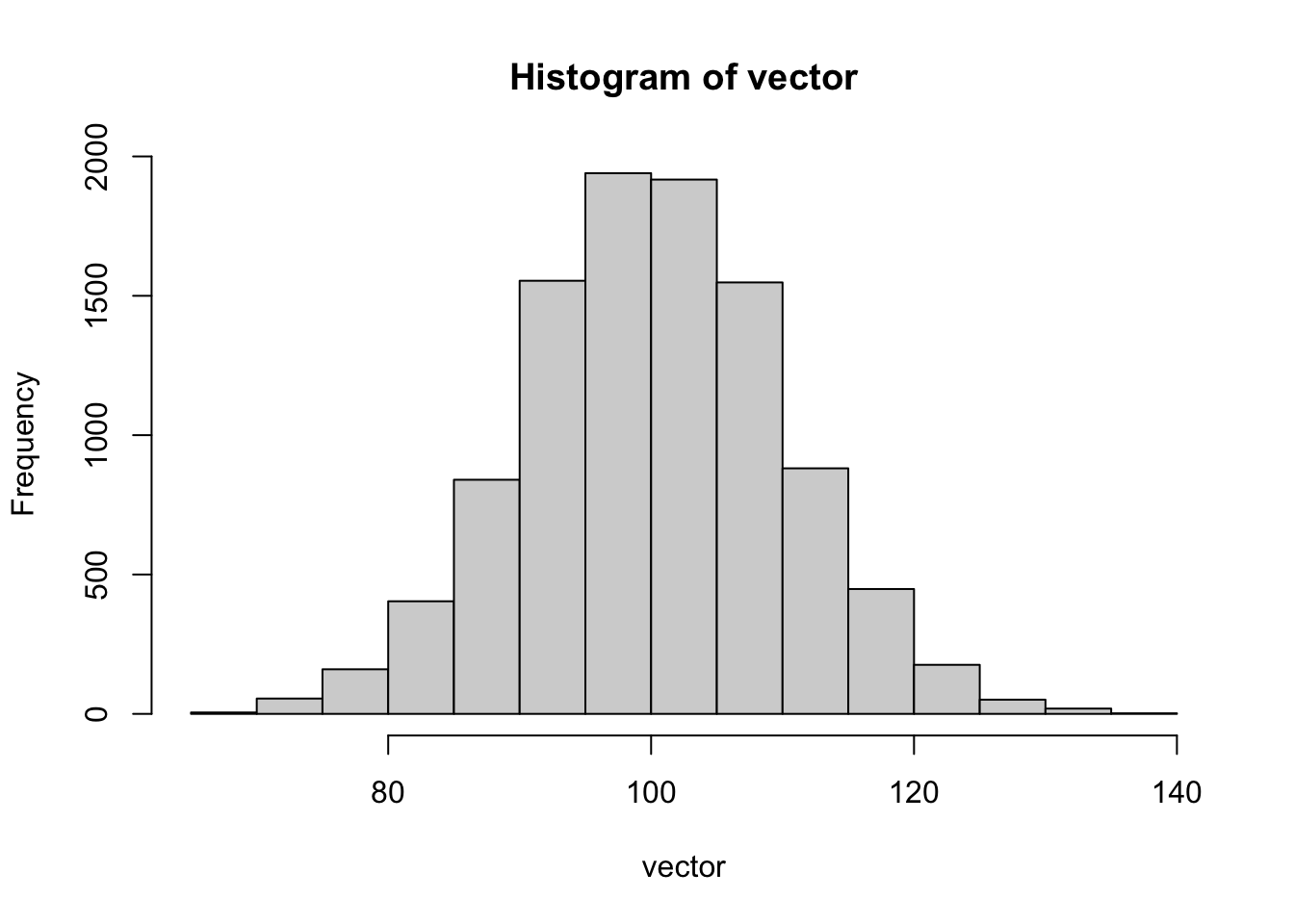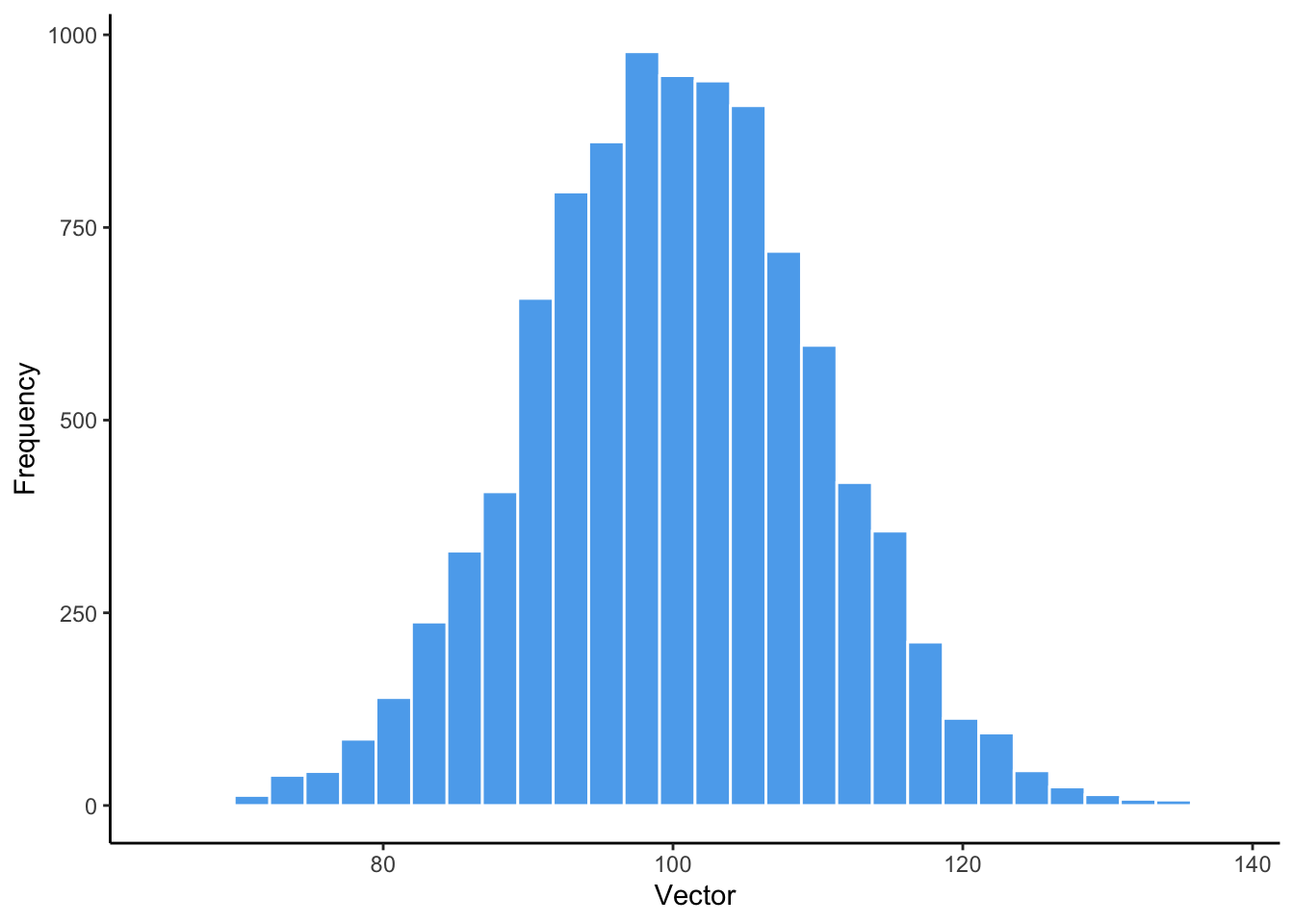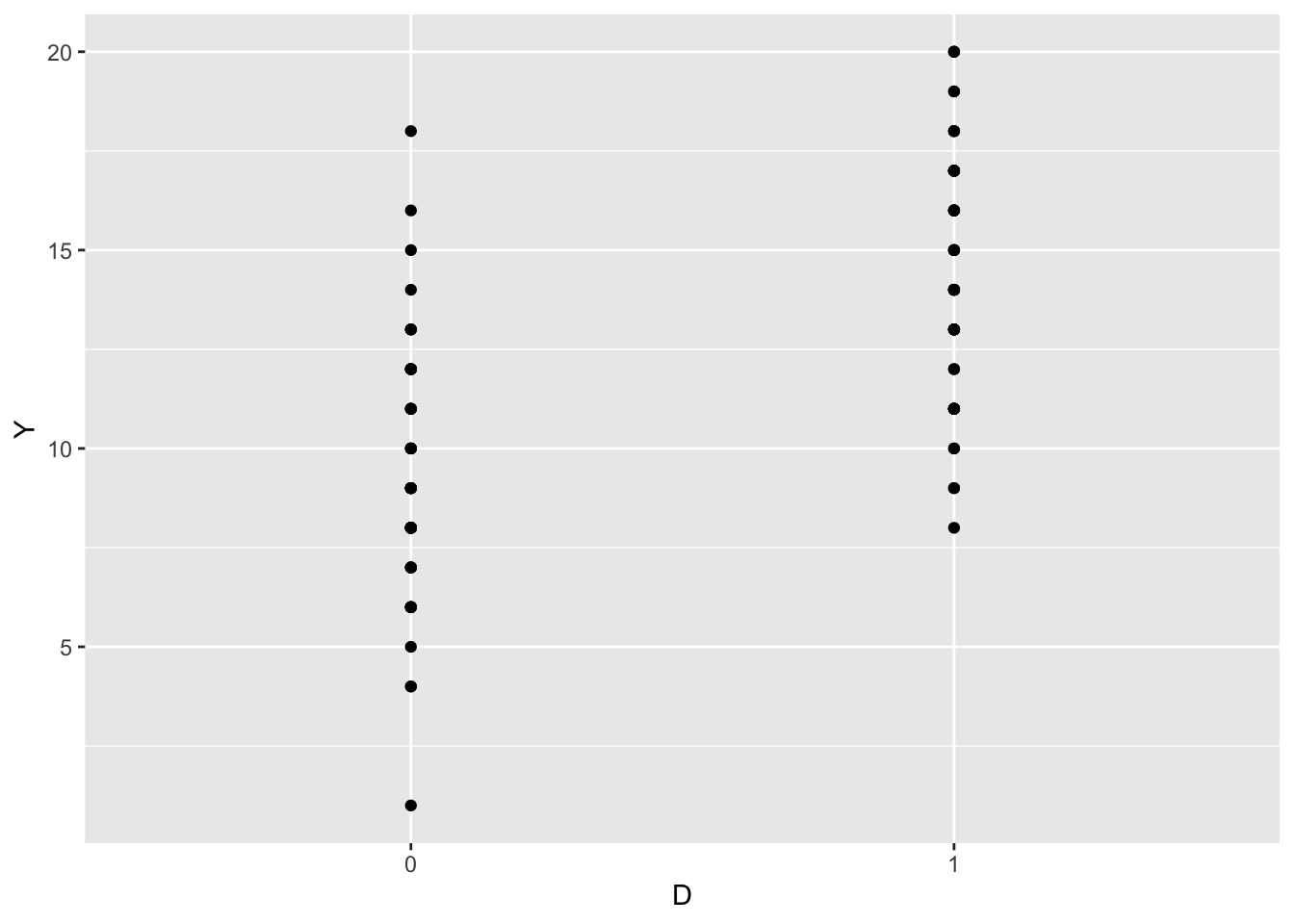Introduction

Officially, the only pre-requisite is any course covering (at any level of detail) linear regression.

This course does not assume prior knowledge of R or a background in statistical computing. However, the learning curve is steep. For example, by week two you will need to be comfortable with loops in R.

You will also be expected to submit your problem sets using rmarkdown.

The primary purpose of these sections is therefore to assist you in gaining the computational skills necessary to understand the material, complete your problem sets, and (most importantly) implement experiments in the real world.

Terminology

Estimand, estimator, and estimate

• Estimand: our quantity of interest.
• Estimator: Statistical procedure (i.e. method) used to make a guess about a parameter. This is a sample statistic, and therefore a random variable. We use the “hat” ($$\hat{\theta}$$ vs. $$\theta$$) symbol to denote an estimator.
• Estimate: the value of the estimand that results from use of a particular estimator (i.e. the value the estimator takes on).

Below are some useful R preamble functions and libraries that we will use in class regularly. Note that before beginning a new work session or starting or working on a new script, you are stronly encouraged to restart your R session.

The tidyverse is a collection of R packages designed for data science. All packages share an underlying design philosophy, grammar, and data structures.

Knitr is a package for producing dynamic reports (e.g. PDFs) directly with R.

KableExtra is a package that builds flxibile and beautiful tables in R.

randomizr is designed to make conducting field, lab, survey, or online experiments easier by automating the random assignment process.

Importing data

First, we are going to import data from a CSV file.

## # A tibble: 20 x 4
##       X1    X2   Yi0   Yi1
##    <dbl> <dbl> <dbl> <dbl>
##  1     0     0     6    17
##  2     0     0     9    12
##  3     1     1     8    17
##  4     1     0    14    13
##  5     1     0    15    14
##  6     1     1     5    15
##  7     0     1    10    13
##  8     1     0     8    16
##  9     1     1     8    16
## 10     0     0    11    15
## 11     0     0     7    15
## 12     0     1     5    16
## 13     1     0     8    13
## 14     1     1    10     9
## 15     1     0    13    19
## 16     0     1    11    11
## 17     0     0    10    16
## 18     1     0     8    12
## 19     1     0     6    18
## 20     1     1     4    19

Data cleaning and manipulation in dplyr

Next we will perform some basic data cleaning and manipulation functions using dplyr.

There are many, many more useful functions in dplyr that are not shown here. Consult “R for Data Science” for a comprehensive overview. If you think a function might exist, or would like to manipulate your data in a particular way but don’t know how, try Googling “how to X in dplyr”!

## # A tibble: 100 x 2
##      Yi1   Yi0
##    <dbl> <dbl>
##  1    17     6
##  2    12     9
##  3    17     8
##  4    13    14
##  5    14    15
##  6    15     5
##  7    13    10
##  8    16     8
##  9    16     8
## 10    15    11
## # … with 90 more rows
## # A tibble: 100 x 2
##      Yi0   Yi1
##    <dbl> <dbl>
##  1     6    17
##  2     9    12
##  3     8    17
##  4    14    13
##  5    15    14
##  6     5    15
##  7    10    13
##  8     8    16
##  9     8    16
## 10    11    15
## # … with 90 more rows
## # A tibble: 100 x 5
##       X1    X2   Yi0   Yi1    X3
##    <dbl> <dbl> <dbl> <dbl> <dbl>
##  1     0     0     6    17     1
##  2     0     0     9    12     1
##  3     1     1     8    17     1
##  4     1     0    14    13     1
##  5     1     0    15    14     1
##  6     1     1     5    15     1
##  7     0     1    10    13     1
##  8     1     0     8    16     1
##  9     1     1     8    16     1
## 10     0     0    11    15     1
## # … with 90 more rows
## # A tibble: 100 x 5
##       X1    X2    X3   Yi0   Yi1
##    <dbl> <dbl> <dbl> <dbl> <dbl>
##  1     0     0     1     6    17
##  2     0     0     1     9    12
##  3     1     1     1     8    17
##  4     1     0     1    14    13
##  5     1     0     1    15    14
##  6     1     1     1     5    15
##  7     0     1     1    10    13
##  8     1     0     1     8    16
##  9     1     1     1     8    16
## 10     0     0     1    11    15
## # … with 90 more rows

Random assignment using randomizr and the switching equation

The code below uses the randomizer package to perform simple and complete random assignment.

Simple random assignment assigns all subjects to treatment with an equal probability (e.g., by flipping a (weighted) coin for each subject). Why might simple random assignment not be the best idea?

• Answer: A different number of subjects might be assigned to each group (e.g., 40 to treatment and 60 to control with N = 100). The number of subjects assigned to treatment is itself a random number.

Complete random assignment allows the researcher to specify exactly how many units are assigned to each condition (e.g., 50 to treatment and 50 to control with N = 100).

Now let’s do something a bit more complex and combine pipes in dplyr with randomizr. We will do the following:

1. Create a vector of randomly assigned treatment statuses.
2. Create a treatment indicator variable $$D$$ based on this vector of treatment assignments.
3. Create a variable of revealed potential outcomes $$Y$$ using the switching equation.
4. Acknowledge the fundamental problem of causal inference by removing the original $$Y_i(0)$$ and $$Y_i(1)$$ variables from our dataset.
5. Calculate the average treatment effect across all units in the sample:

($$E[Y_i(1) | D = 1] - E[Y_i(0) | D = 0]$$).

## # A tibble: 100 x 3
##        D     Y   ATE
##    <int> <dbl> <dbl>
##  1     0     6   5.2
##  2     0     9   5.2
##  3     1    17   5.2
##  4     1    13   5.2
##  5     1    14   5.2
##  6     0     5   5.2
##  7     1    13   5.2
##  8     1    16   5.2
##  9     0     8   5.2
## 10     0    11   5.2
## # … with 90 more rows

We could also calculate treatment effects for indvidual units here, but this is a completely hypothetical exercise. Why?

• Answer: In reality, we can never see an individual unit’s treatment and control potential outcomes. If a unit is treated, it reveals its treatment outcome. If it is in control, it reveals its control outcome. This fact—that it is impossible to observe the causal effect on a single unit—is known as the Fundamental Problem of Causal Inference.

## # A tibble: 100 x 3
##      Yi1   Yi0  diff
##    <dbl> <dbl> <dbl>
##  1    17     6    11
##  2    12     9     3
##  3    17     8     9
##  4    13    14    -1
##  5    14    15    -1
##  6    15     5    10
##  7    13    10     3
##  8    16     8     8
##  9    16     8     8
## 10    15    11     4
## # … with 90 more rows

Group by and summarise

Now we will use another particularly useful combination of dplyr functions often refered to as “group by summarise.” This allows us to calculate a number of summary statistics for a given dataframe/tibble.

Let’s display the output of our function in a pretty manner using Kable. Note that Kable has many more functions for customizing tables for output to both PDF and HTML that you can explore.

D groupmean size var se ATE
0 9.2 50 10.285714 0.4535574 5.2
1 14.4 50 9.795918 0.4426267 5.2

Loops

Loops allow you to reduce duplication in your code by repeating a function iteratively. This helps you when you need to do the same thing to multiple inputs, for example repeating the same operation on different rows, columns, or on different datasets.

As a general rule of thumb, you should not copy and past the same line of code to repeat a function more than two or three times, but there will also be times when you need to repeat a function more times than is practically possible by copying lines of code. These kinds of operations are often essential for random sampling and simulation, and therefore experiments.

First, we will practice a basic loop where we create a empty vector and fill it with random draws from a normal distribution. You can create “for loops,” “while loops,” and “repeat loops” in R. For this class you will need a firm understanding of “for loops.”

Next, we will create a plot of this thing using base R plotting. Note that I am only showing you base R plots so that you can see how much we can improve on this with ggplot in a moment!Next week we will practice a procedure for hypothesis testing called randomization inference. This will require you to have a firm understanding of loops, so please ensure that you do, or come to office hours if anything is confusing!

ggplot2

Now we will create a plot using ggplot2—arguably the best and most flexible data visualization platform around. Other languages and packages have sophisticated plotting functions (e.g., Python’s Seaborn, Stata’s plotting functions, Tableau, etc.), but none are as comprehensive, flexible, and developed as ggplot2.

First, let’s recreate the histogram above using ggplot2.Now let’s go step by step through how ggplot works, and construct a visualization of the average treatment effect we calculated above.

ggplot elements can be added iteratively in layers. In other words, you can continually add new elements to or on top of a plot. Let’s start by creating a basic scatterplot of our outcome variable $$Y$$ versus our treatment indicator $$D$$.That’s a bit weird? We have 50 observations in each treatment group, but only 15 observations appear in control and 13 in treatment on the plot. This is because our points are on top of each other, we can give them some wiggle room using the “geom_jitter” function.